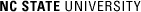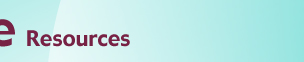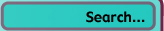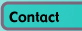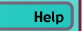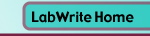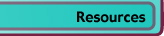# Graphing Resources

## Using Error Bars in your Graph

The knowledge that any individual measurement you make in a lab will lack perfect precision often leads a researcher to choose to take multiple measurements at some independent variable level. Though no one of these measurements are likely to be more precise than any other, this group of values, it is hoped, will cluster about the true value you are trying to measure. This distribution of data values is often represented by showing a single data point, representing the mean value of the data, and error bars to represent the overall distribution of the data.

Let's take, for example, the impact energy absorbed by a metal at various temperatures. In this case, the temperature of the metal is the independent variable being manipulated by the researcher and the amount of energy absorbed is the dependent variable being recorded. Because there is not perfect precision in recording this absorbed energy, five different metal bars are tested at each temperature level. The resulting data (and graph) might look like this: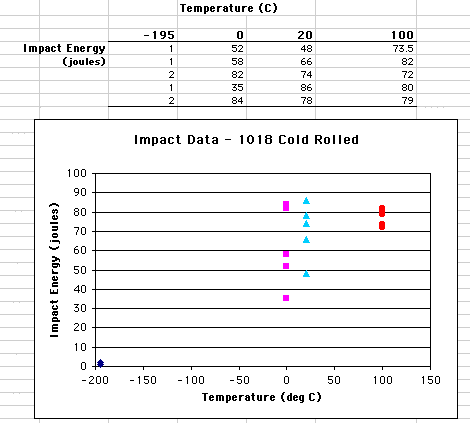For clarity, the data for each level of the independent variable (temperature) has been plotted on the scatter plot in a different color and symbol. Notice the range of energy values recorded at each of the temperatures. At -195 degrees, the energy values (shown in blue diamonds) all hover around 0 joules. On the other hand, at both 0 and 20 degrees, the values range quite a bit. In fact, there are a number of measurements at 0 degrees (shown in purple squares) that are very close to measurements taken at 20 degrees (shown in light blue triangles). These ranges in values represent the uncertainty in our measurement. Can we say there is any difference in energy level at 0 and 20 degrees? One way to do this is to use the descriptive statistic, mean.

The mean, or average, of a group of values describes a middle point, or central tendency, about which data points vary. Without going into detail, the mean is a way of summarizing a group of data and stating a best guess at what the true value of the dependent variable value is for that independent variable level. In this example, it would be a best guess at what the true energy level was for a given temperature. The above scatter plot can be transformed into a line graph showing the mean energy values: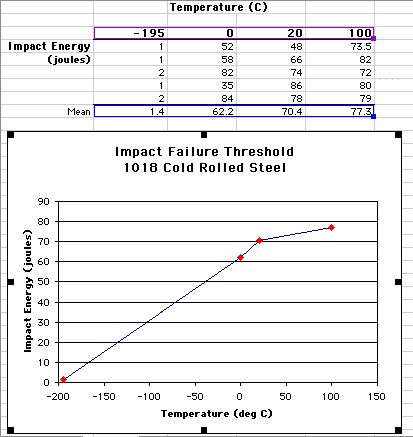Note that instead of creating a graph using all of the raw data, now only the mean value is plotted for impact energy. The mean was calculated for each temperature by using the AVERAGE function in Excel. You use this function by typing =AVERAGE in the formula bar and then putting the range of cells containing the data you want the mean of within parentheses after the function name, like this: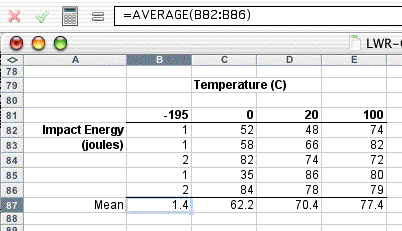In this case, the values in cells B82 through B86 are averaged (the mean calculated) and the result placed in cell B87. Once you have calculated the mean for the -195 values, then copy this formula into the cells C87, etc. If you look back at the line graph above, we can now say that the mean impact energy at 20 degrees is indeed higher than the mean impact energy at 0 degrees. However, though you can say that the means of the data you collected at 20 and 0 degrees are different, you can't say for certain the true energy values are different. Can we ever know the true energy values? No, but you can include additional information to indicate how closely the means are likely to reflect the true values. You can do this with error bars.

There are two common ways you can statistically describe uncertainty in your measurements. One is with the standard deviation of a single measurement (often just called the standard deviation) and the other is with the standard deviation of the mean, often called the standard error. Since what we are representing the means in our graph, the standard error is the appropriate measurement to use to calculate the error bars. While we were able to use a function to directly calculate the mean, the standard error calculation is a little more round about. First you have to calculate the standard deviation with the STDEV function. It is used much the same way AVERAGE was: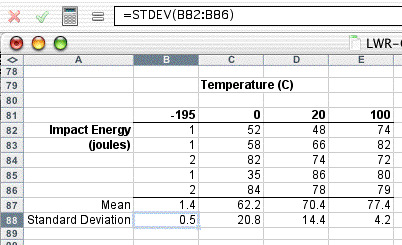The standard error is calculated by dividing the standard deviation by the square root of number of measurements that make up the mean (often represented by N). In this case, 5 measurements were made (N = 5) so the standard deviation is divided by the square root of 5. By dividing the standard deviation by the square root of N, the standard error grows smaller as the number of measurements (N) grows larger. This reflects the greater confidence you have in your mean value as you make more measurements. You can make use of the of the square root function, SQRT, in calculating this value: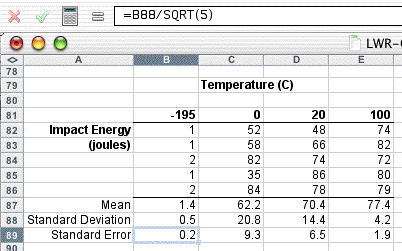Using words you can state that, based on five measurements, the impact energy at -195 deg C is 1.4 +/- 0.2 joules. The +/- value is the standard error and expresses how confident you are that the mean value (1.4) represents the true value of the impact energy. Graphically you can represent this in error bars.

With the standard error calculated for each temperature, error bars can now be created for each mean. First click the line in the graph so it is highlighted. Now select Format>Selected Data Series...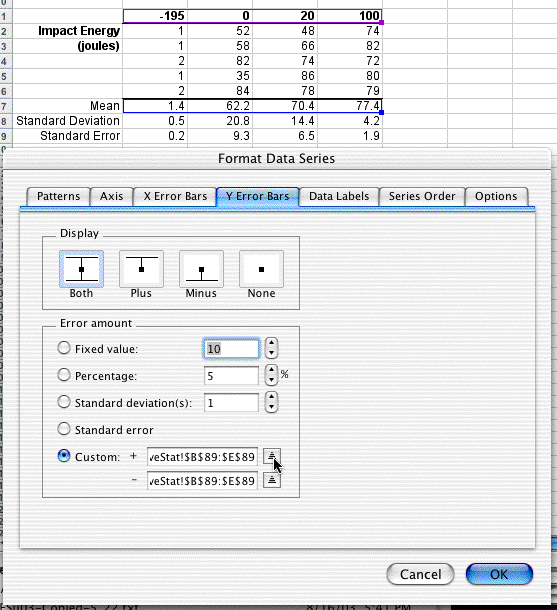Select the Y Error Bars tab and then choose to Display Both (top and bottom error bars). Now click on the Custom button as the method for entering the Error amount. You will want to use the standard error to represent both the + and the - values for the error bars, B89 through E89 in this case. Note: it is critical to highlight the standardard deviation values for all of the temperatures. This way the unique standard error value is associated with each mean. The easiest way to do this is to click on the up arrow button as shown in the figure above. The dialog box will now shrink and allow you to highlight cells representing the standard error values: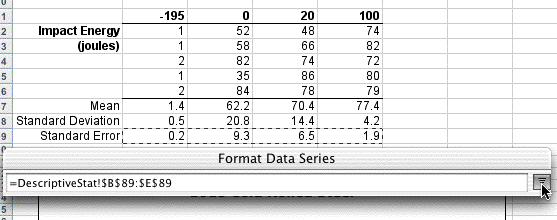When you are done, click on the down arrow button and repeat for the other value cell. When you are done, click OK. Your graph should now look like this: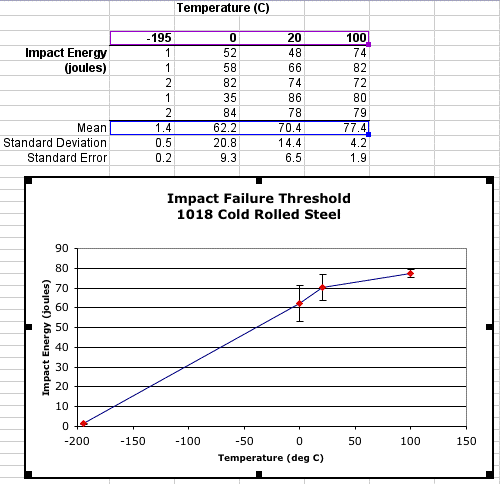The error bars shown in the line graph above represent a description of how confident you are that the mean represents the true impact energy value. The more the orginal data values range above and below the mean, the wider the error bars and less confident you are in a particular value. Compare these error bars to the distribution of data points in the original scatter plot above.Tight distribution of points around 100 degrees - small error bars; loose distribution of points around 0 degrees - large error bars. More precisely, the part of the error bar above each point represents plus one standard error and the part of the bar below represents minus one standard error.

With the error bars present, what can you say about the difference in mean impact values for each temperature? If the upper error bar for one temperature overlaps the range of impact values within the error bar of another temperature, there is a much lower likelihood that these two impact values differ significantly. Therefore, we can say with some confidence that the impact energy at 0, 20, and 100 degrees is significantly greater than at -195 degrees. We can also say the same of the impact energy at 100 degrees from 0 degrees. However, we are much less confident that there is a significant difference between 20 and 0 degrees or between 20 and 100 degrees. How can we improve our confidence? One way would be to take more measurements and shrink the standard error. However, remember that the standard error will decrease by the square root of N, therefore it may take quite a few measurements to decrease the standard error. It is also possible that your equipment is simply not sensitive enough to record these differences or, in fact, there is no real significant difference in some of these impact values.

If you are also going to represent the data shown in this graph in a table or in the body of your lab report, you may want to refer to the resources on significant digits and designing tables.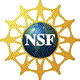© Copyright NC State University 2004 Sponsored and funded by National Science Foundation (DUE-9950405 and DUE-0231086) Site design by Rosa Wallace Rev. RW 5/16/05